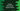# Python program to find out the largest divisor of a number## Find the largest divisor using Python:

In this tutorial, we will learn how to find out the largest divisor of a number in python. The largest divisor of a number is the largest number that can divide it. It should not be the number itself. For example, for number 10, it can be divided by 1,2,5 and 10. So, the largest divisor is 5.

To solve this problem, first of all, we will ask the user to enter a number. Then we will use one loop to check each number if it can divide the user input number. If yes, it will be considered as the largest divisor until the loop is completed.

Let’s take a look at the program to understand how it works :

### Python program :

``````#1
num = int(input("Enter a number : "))
largest_divisor = 0

#2
for i in range(2, num):
#3
if num % i == 0:
#4
largest_divisor = i

#5
print("Largest divisor of {} is {}".format(num,largest_divisor))``````

### Explanation :

The commented numbers in the above program denote the step numbers below:

1. Ask the user to enter a number. Read the number as an integer using the int() function and save it in num variable. Also, create one more variable largest_divisor to store the largest divisor for the user input number.
2. Run one for loop from 2 to the user input number.
3. For each number in the loop, check if it can divide the user input number or not.
4. If the number can divide the user input number, assign it to largest_divisor variable.
5. After the for loop will complete, the largest_divisor variable will hold the largest divisor for the user input number. Print it out.

### Sample output :

``````Enter a number : 50
Largest divisor of 50 is 25

Enter a number : 112
Largest divisor of 112 is 56

Enter a number : 10
Largest divisor of 10 is 5

Enter a number : 50
Largest divisor of 50 is 25``````

This program is available on Github.

### Conclusion :

We have learned how to find out the largest divisor of a number in python. We are using one for loop to find out the largest divisor in this example. But you can also use one while loop instead. Try to run the above examples and drop one comment below if you have any queries.